# A bag

A bag contains 9 blue marbles and 1 green marble. What is the probability of drawing a blue marble followed by a green marble, without replacing the first marble before drawing the second marble.

p =  0.1

### Step-by-step explanation: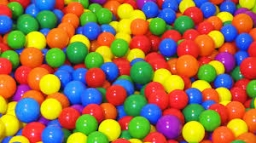Did you find an error or inaccuracy? Feel free to write us. Thank you!Tips to related online calculators
Need help to calculate sum, simplify or multiply fractions? Try our fraction calculator.
Would you like to compute count of combinations?

## Related math problems and questions:

• Marble bagA marble bag sold by Rachel's Marble Company contains 5 orange marbles for every 6 green marbles. If a bag has 35 orange marbles, how many green marbles does it contain?
• In PEIn PE, students play a game where they do different exercises depending on the color of marble that Coach Forbes draws. Coach Forbes has a jar with 6 red marbles, 12 blue marbles, 16 purple marbles, 2 green marbles, and 4 yellow marbles. What is the proba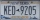A license plate has 3 letters followed by 4 numbers. Repeats are not allowed for the letters, but they are for the numbers. If they are issued at random, what is the probability that the 3 letters are in alphabetical order and the 3 numbers are consecutiv
• Components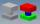In the box are 8 white, 4 blue and 2 red components. What is the probability that we pull one white, one blue and one red component without returning?
• Drawing from a hatWhen drawing numbers from a hat from 1 to 35, we select random given numbers. What is the probability that the drawn numbers will be divisible by 8 and 2?
• A boxA box contains 6 red balls, 5 blue, and 4 white balls. Find the probability of drawing a) red ball b) non-white ball.
• Green - Red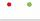We have 5 bags. Each consist one green and 2 red balls. From each we pull just one ball. What is the probability that we doesn't pull any green ball?
• Red ballsIn the bag there are 3 red, 12 blue and 8 green balls. How many red balls we must be attached to the bag if we want the probability of pulling out the red balls was 20%?
• DicesWe will throw two dice. What is the probability that the ratio between numbers on first and second dice will be 1:2?
• Coloured numbersMussel wrote four different natural numbers with coloured markers: red, blue, green and yellow. When the red number divides by blue, it gets the green number as an incomplete proportion, and yellow represents the remainder after this division. When it div
• Balls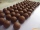From the bag with numbered balls (numbers 1,2,3,. ..20) we pick one ball. What is the probability of choosing a number containing 1?
• Karolína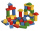Karolína chose 5 bodies from the kit - white, blue and gray cubes, a blue cylinder and a white triangular prism. How many different roof towers can be built one by one if all the blue bodies (cube and cylinder) are not placed on top of each other?
• One threeWe throw two dice. What is the probability that max one three falls?
• Tricolors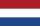From the colors - red, blue, green, black, and white, create all possible tricolors.
• ThrowWe throw 2 times with 2 dices. What is the probability that the first roll will fall more than sum of 9 and the second throw have sum 3 or does not have the sum 4?
• We rollWe roll two dice A. - what is the probability that the sum of the falling numbers is at most 4 B. - is at least 10 C. - is divisible by 5?
• There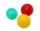There are two numbers on the screen - one in the blue and the other in the red box. In the beginning, both numbers are the same. With each beep, both numbers increase - by 1 in the blue field and by 3 in the red field. At one point, the number 49 appears